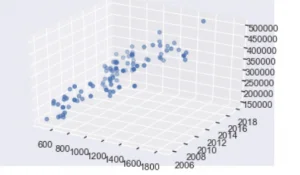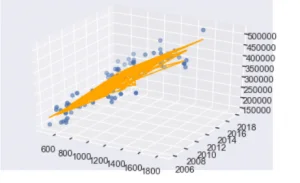14 Oct 2019

Posted on:

07 Oct 2019

0

# Resolved:How to scatter plot the real estate document with 2 independent variables (size, year)

From Machine Learning - Multiple Linear Regression - Using Python 3, how can I create a scatter plot given 2 independent variables (year, size)?  And what would the linear regression formula be?
Instructor
Posted on:

08 Oct 2019

1

Hi Casey,
You know that if you have a single predictor, then you'd have a 2D plot, right? There's 1 independent X and the a dependent Y (say 'size' and 'price' in that case).
To plot 2 independent variables, you will need yet another dimension. In that case you will have a 3D plot. To achieve that you can use the mplot3d package (built on top of matplotlib). Here's the documentation: https://matplotlib.org/mpl_toolkits/mplot3d/
So, assuming that your 2 independent variables are 'size' and 'year', while the dependent is 'price', for the specific exercise you are referring to, you can use code in the lines of:

`from mpl_toolkits.mplot3d import axes3d, Axes3Dfig = plt.figure()ax = plt.axes(projection="3d")ax.scatter3D(data['size'], data['year'], data['price'], cmap='hsv')plt.show()`

This will result into a 3D scatter plot:Now what if you want to plot a regression line?
First, it is important to note that it is a regression line, in 2D, however, in 3D that would be a plane.
To find that plane with statsmodels (and within the given example), we can write:

`results.params + results.params*data['size'] + results.params*data['year']`

Note that here, results.params contains the coefficients of the regression. There are 3 of them: constant, coef. for size and coef. for year. So the regression line becomes the abovementioned equation. Finally, we can incorporate that in the graph, using a 3D line (plane).

`from mpl_toolkits.mplot3d import axes3d, Axes3Dfig = plt.figure()ax = plt.axes(projection="3d") x_line = data['size']y_line = data['year']z_line = results.params + results.params*data['size'] + results.params*data['year']ax.plot3D(x_line, y_line, z_line, 'orange')ax.scatter3D(data['size'], data['year'], data['price'], cmap='hsv')plt.show()`

And what you'll see is:Best,
The 365 Team

Posted on:

09 Oct 2019

0

Ok, I am almost there, thank you, but the following code displayed the same looking plane with no dots.  What's a scatter plot with no dots?!  Any ideas on why the dots are missing?

`fig = plt.figureax = plt.axes(projection="3d") x_line = data['size']y_line = data['year']z_line = results.params + results.params*data['size'] + results.params*data['year']ax.plot3D(x_line, y_line, z_line, 'orange')plt.show()`

Instructor
Posted on:

09 Oct 2019

0

Hi Casey,
The scatter itself is created with the code:

`ax.scatter3D(data['size'], data['year'], data['price'], cmap='hsv')`

I believe you are missing this bit. This scatter represents the points. The rest of the code (the one you've implemented) draws the regression line. To get only the scatter, please refer to the first code cell in the original answer:

`fig = plt.figure()ax = plt.axes(projection="3d")ax.scatter3D(data['size'], data['year'], data['price'], cmap='hsv')plt.show()`
`Hope this helps,The 365 Team`
Posted on:

09 Oct 2019

0

`from mpl_toolkits.mplot3d import axes3d, Axes3D`

Posted on:

09 Oct 2019

0

So I tried this because the code following the # leads to an IndexError: Index is out of bounds. Can you explain why that is?  Also, the code below yields a different plane than yours, though I don't know how to post it

``````fig = plt.figure()
ax = plt.axes(projection="3d")
x_line = data['size']
y_line = data['year']
z_line = data['price']
#z_line = results.params + results.params*data['size'] + results.params*data['year']
ax.plot3D(x_line, y_line, z_line, 'orange')
ax.scatter3D(data['size'], data['year'], data['price'], cmap='hsv')
plt.show()
``````
Posted on:

12 Oct 2019

0
I don't know, I'm having trouble capturing a screenshot of my work
Posted on:

14 Oct 2019

0

I posted it to imgur.com but I don't know how you'll find it so I embedded it
Multiple Linear Regression

Posted on:

14 Oct 2019

0